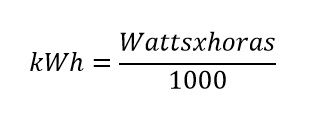# Watts to kWh – Convert, conversion, equivalence, table and formula

With this tool you can convert online from Watts to kWh automatically, easy, fast and free.

For greater ease we explain that formula is used for the calculation and a table with the main conversions of Watts to kWh.

## Definitions P (Watts) and kWh:

Kwh = The kilowatt-hour (symbol kWh), is the power in kilo watts multiplied by the time in hours. The kilowatt-hour is commonly used as a power billing unit supplied to consumers by power companies.

P (Watts) = Called active power, it is the power capable of transforming electrical energy into work. The different electrical devices existing convert electrical energy into other forms of energy such as: mechanical, light, thermal, chemical, etc. This power is therefore the actual consumed by the circuits and, consequently, when talking about demand, it is this power that is used to determine such demand.

## Formula to convert, calculate, transform Watts to kWh:## Table for conversion, equivalence, transformation from Watts to kWh:

 Watts Hr kwh 1 watts 1 Hr 0,001 kwh 2 watts 1 Hr 0,002 kwh 3 watts 1 Hr 0,003 kwh 4 watts 1 Hr 0,004 kwh 5 watts 1 Hr 0,005 kwh 6 watts 1 Hr 0,006 kwh 7 watts 1 Hr 0,007 kwh 8 watts 1 Hr 0,008 kwh 9 watts 1 Hr 0,009 kwh 10 watts 1 Hr 0,01 kwh 20 watts 2 Hr 0,04 kwh 30 watts 2 Hr 0,06 kwh 40 watts 2 Hr 0,08 kwh 50 watts 2 Hr 0,1 kwh 60 watts 2 Hr 0,12 kwh 70 watts 2 Hr 0,14 kwh 80 watts 2 Hr 0,16 kwh 90 watts 2 Hr 0,18 kwh 100 watts 2 Hr 0,2 kwh 200 watts 3 Hr 0,6 kwh 300 watts 3 Hr 0,9 kwh 400 watts 3 Hr 1,2 kwh 500 watts 3 Hr 1,5 kwh 600 watts 3 Hr 1,8 kwh 700 watts 3 Hr 2,1 kwh 800 watts 3 Hr 2,4 kwh 900 watts 3 Hr 2,7 kwh 1000 watts 3 Hr 3 kwh 2000 watts 4 Hr 8 kwh 3000 watts 4 Hr 12 kwh 4000 watts 4 Hr 16 kwh 5000 watts 4 Hr 20 kwh 6000 watts 4 Hr 24 kwh 7000 watts 4 Hr 28 kwh 8000 watts 4 Hr 32 kwh 9000 watts 4 Hr 36 kwh 10000 watts 4 Hr 40 kwh 20000 watts 5 Hr 100 kwh 30000 watts 5 Hr 150 kwh 40000 watts 5 Hr 200 kwh 50000 watts 5 Hr 250 kwh 60000 watts 5 Hr 300 kwh 70000 watts 5 Hr 350 kwh 80000 watts 5 Hr 400 kwh 90000 watts 5 Hr 450 kwh 100000 watts 5 Hr 500 kwh

Rate this calculator Watts to kWH:  [kkstarratings]